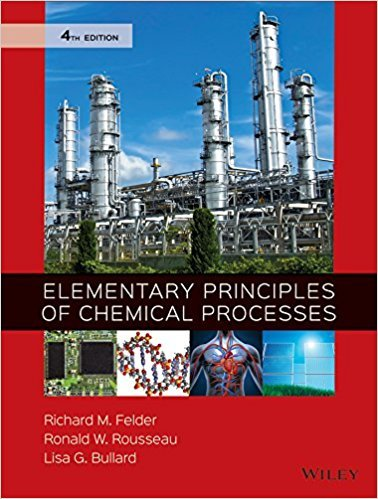×
×

# Solutions for Chapter 4.108: SUMMARY## Full solutions for Elementary Principles of Chemical Processes | 4th Edition

ISBN: 9780470616291Solutions for Chapter 4.108: SUMMARY

Solutions for Chapter 4.108
4 5 0 379 Reviews
13
0
##### ISBN: 9780470616291

Elementary Principles of Chemical Processes was written by and is associated to the ISBN: 9780470616291. This textbook survival guide was created for the textbook: Elementary Principles of Chemical Processes, edition: 4. This expansive textbook survival guide covers the following chapters and their solutions. Since 1 problems in chapter 4.108: SUMMARY have been answered, more than 41489 students have viewed full step-by-step solutions from this chapter. Chapter 4.108: SUMMARY includes 1 full step-by-step solutions.

Key Chemistry Terms and definitions covered in this textbook
• alkynes.

Hydrocarbons that contain one or more carbon-carbon triple bonds. They have the general formula CnH2n22, where n 5 2,3, . . . . (24.2)

• binary compounds.

Compounds formed from just two elements. (2.7)

• boiling point.

The temperature at which the vapor pressure of a liquid is equal to the external atmospheric pressure. (11.8)

• Brønsted-Lowry base

A proton acceptor

• coal

A naturally occurring solid containing hydrocarbons of high molecular weight, as well as compounds containing sulfur, oxygen, and nitrogen. (Section 5.8)

• combination reaction

A chemical reaction in which two or more substances combine to form a single product. (Section 3.2)

• condensation reaction.

A reaction in which two smaller molecules combine to form a larger molecule. Water is invariably one of the products of such a reaction. (24.4)

The magnitude of charge of one mole of electrons: 96,500 C>mol. (Section 20.5)

• hydrolysis

A reaction with water. When a cation or anion reacts with water, it changes the pH. (Sections 16.9 and 24.4)

• net ionic equation

A chemical equation for a solution reaction in which soluble strong electrolytes are written as ions and spectator ions are omitted. (Section 4.2)

• node

In atomic and molecular orbitals, a location where the value of y is zero.

• norbornane

The common name for bicyclo[2.2.1]heptane.

• pi 1P2 bond

A covalent bond in which electron density is concentrated above and below the internuclear axis. (Section 9.6)

• Quaternary (4°) ammonium ion

An ion in which nitrogen is bonded to four carbons and bears a positive charge

• s-cis

A conformation of a conjugated diene in which the disposition of the two p bonds with regard to the connecting single bond is cis-like (a dihedral angle of 0°).

• Sharpless asymmetric epoxidation

A reaction that converts an alkene into an epoxide via a stereospecific pathway.

• solution

A mixture of substances that has a uniform composition; a homogeneous mixture. (Section 1.2)

• spin magnetic quantum number 1ms2

A quantum number associated with the electron spin; it may have values of +12 or -12. (Section 6.7)

• Telechelic polymer

A polymer in which its growing chains are terminated by formation of new functional groups at both ends of its chains. These new functional groups are introduced by adding reagents, such as CO2 or ethylene oxide, to the growing chains.

• wavelength

The distance between adjacent peaks of an oscillating magnetic or electric field.

×